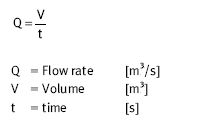﻿ Flow rate – Hydraulic Schematic Troubleshooting

## Flow rate

Flow rate is the term used to describe the volume of liquid flowing through a pipe in a specific period of time. For example, approximately one minute is required to fill a 10 litre bucket from a tap. Thus, the flow rate amounts to 10 l/min.

In hydraulics, the flow rate is designated as Q. The following equation applies:The equations for the volume (V) and the time (t) can be derived from the formula for the flow rate. The following equation is produced:

V = Q · t